# Temperature dependence in EDFA

Compatibility:

This lesson demonstrates the temperature dependence in the absorption coefficient of an EDFA.
The temperature dependence in an EDFA manifests through the variation in the absorption α and gain g* coefficients (or absorption σa and emission σe cross-sections) , . Here, to demonstrate the effect of temperature dependence, simulations are done using different temperatures and a method to characterize the EDF’s absorption coefficient at each temperature is used.
In the first part of this lesson, the method for calculating the EDF’s absorption coefficient is described. The system shown in Figure 1 is used for the characterization of the absorption coefficient. It consists of a CW laser source with signal power equal -20dBm and wavelength varying at the range of 1450nm to 1650nm (the CW laser is in the sweep mode).
The Optical Power Meter Visualizer gives the output power in the EDFA. The Erbium doped fiber has the physical parameters shown in Figure 2(a) and the original absorption coefficient loaded is shown in Figure 2(b).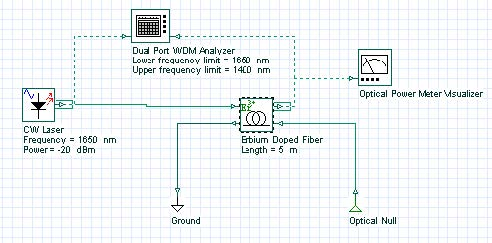Figure 1: System layout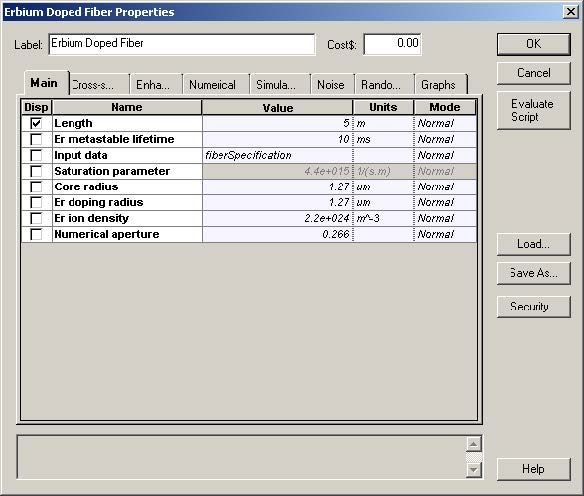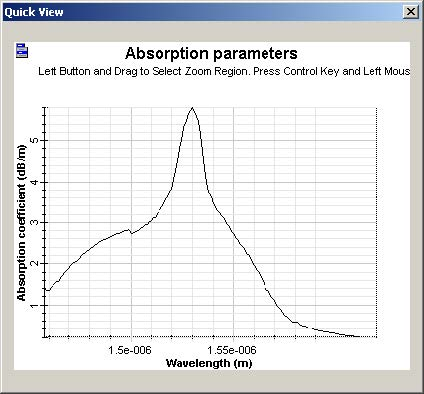Figure 2: (a) Main tab with the parameters used in the simulations (b) Absorption coefficient loaded

The following procedure was used to calculate the absorption coefficient from the data
obtained in simulations. To determine the value of the absorption coefficient of the
EDF, simulations for two fiber lengths (5m and 0.5m) were conducted in the
wavelength range of 1450 to 1650nm. Using the corresponding output signal powers
Pout(λ , L = 5m) and Pout(λ , L = 0.5m), the absorption coefficient can be
obtained by the equation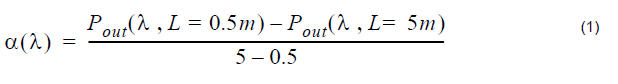In Figure 3 we compare the original absorption coefficient loaded on the EDF and the calculated one using Equation 1. You can see the agreement between the two sets of results. Therefore, this procedure can be used to calculate the absorption coefficient of an EDFA.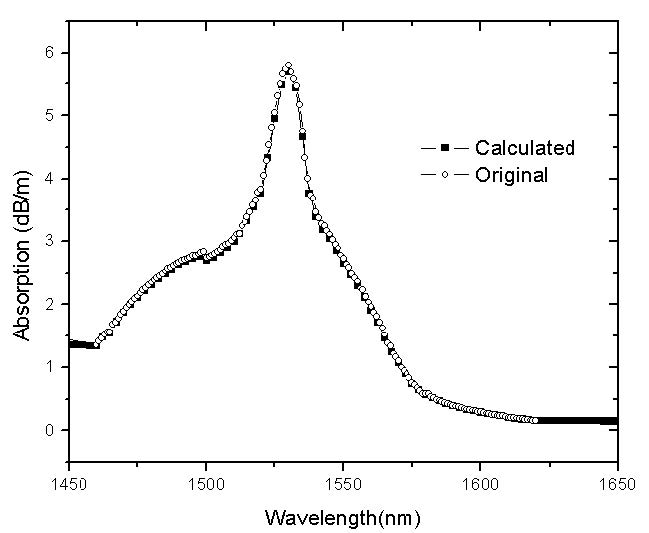Figure 3: Comparison between the original and the calculated absorption coefficient

In the second part of this lesson, the temperature dependence of the absorption coefficient is demonstrated. The method used to calculate the absorption coefficient is used again, but this time, the simulations considered different temperatures. With this procedure, the temperature dependence in the absorption coefficient is demonstrated.

To consider the temperature dependence in the Erbium-doped fiber component, Include Temperature Effect on the Enhanced tab must be selected, and the temperature that the cross sections (loaded) were measured at must be provided.

Here, the original cross sections (Figure 2b) were measured at 20°C. Finally, user the current temperature must be defined in order for it to be considered in the simulation temperature parameter. Figure 4 shows an example using 80°C for the simulation.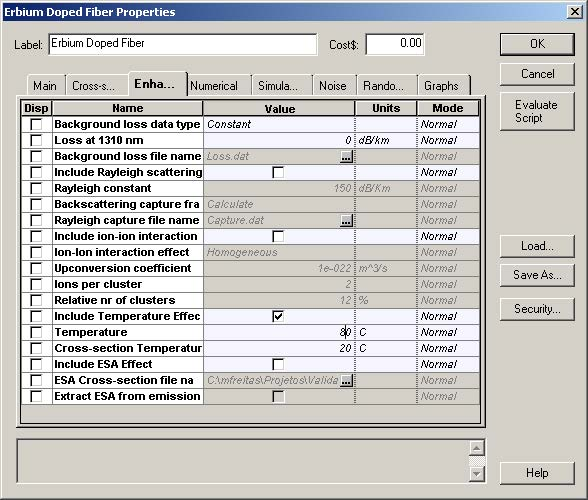Figure 4: Enhanced tab with simulation setup

Simulations were done for three temperatures, -40°C–, + 20°C, and + 80°C. For each temperature, the absorption coefficients were calculated using Equation 1. Figure 5 shows the results for the three temperatures simulated. It is possible to see the differences in the absorption coefficients caused by the changes in temperature.

These results seem in good qualitative agreement with presented studies in . The same approach can be taken to analyze temperature dependence in the gain coefficient. However, the procedure and the system setup used to calculate the gain coefficient will be different, because it will be necessary to find the fluorescence spectrum.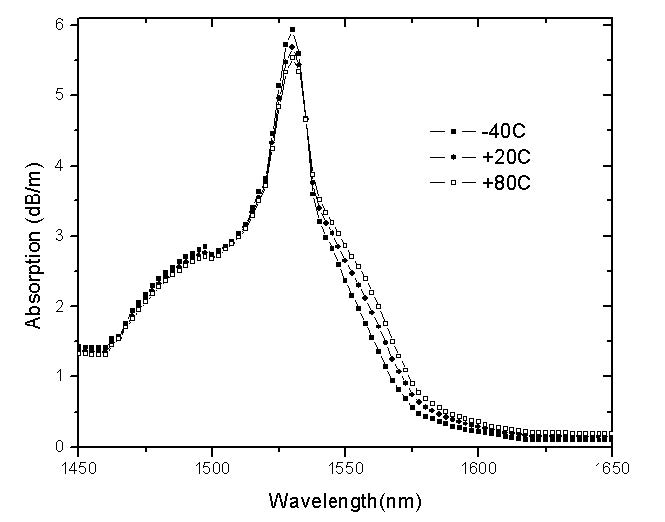Figure 5: Absorption coefficient calculated for different temperatures

This variation in the absorption and gain coefficients causes variation in the performance of the amplifier, gain, and noise was demonstrated in  and .

References:

P. F. Wysocki, N. Conti, and D. Holcomb. “Simple Modeling Approach for the Temperature Dependence of the Gain of Erbium-Doped Fiber Amplifiers”. SPIE Conference on Optical Devices for Fiber Communication, Volume 3847, 1999.

M. Bolshtyansky, P. F. Wysocki, N. Conti. “Model of Temperature Dependence for Gain Shape of Erbium-Doped Fiber”. Journal of Lightwave Technology, volume 18, Issue 11, Dec 2000.